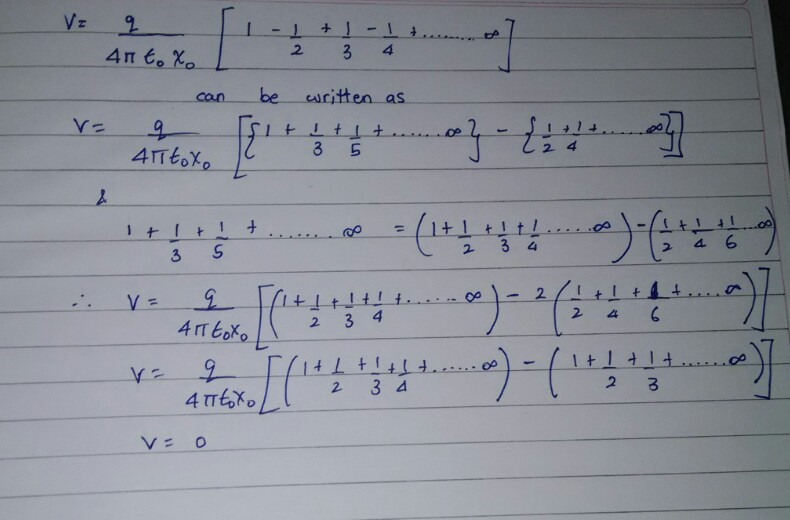# Mathematical Physics Dilemma

Hello everyone.

I came across a physics question as follows,

A set of positive point charges are kept at the points on the x-axis, such that

$$x=x_{0}$$,$$x=3x_{0}$$,$$x=5x_{0}$$........$$\infty$$

And a set of negative point charges are placed on the x-axis, such that,

$x=2x_{0}$,$x=4x_{0}$,$x=6x_{0}$........$\infty$

If the magnitude of the positive point charge is $+q$ and the magnitude of the negative point charge is $-q$, assuming $\varepsilon_0$ is the absolute permittivity of free space, then prove that the electric potential at the origin is

$V=\frac{q\ln2}{4\pi\varepsilon_0x_{0}}$

This is how I proved it.

We know by the definition of electric potential due to a point charge,

$V=\frac{q}{4\pi\varepsilon_0x_{0}}$

where $x_{0}$ is the separation between the origin and the point where the point charge is placed. Also it is known that the electric potential due to a system of point charges is the algebraic sum of the electric potentials due to the individual point charges.

Hence by applying this to every single point charge given, we get

$V=\frac{q}{4\pi\varepsilon_0(x_{0})} + \frac{-q}{4\pi\varepsilon_0(2x_{0})} + \frac{q}{4\pi\varepsilon_0(3x_{0})} + \frac{-q}{4\pi\varepsilon_0(4x_{0})}+.............\infty$

Factoring out $\frac{q}{4\pi\varepsilon_0x_{0}}$, we get

$V=\frac{q}{4\pi\varepsilon_0(x_{0})}[1-\frac{1}{2}+\frac{1}{3}-\frac{1}{4}+.............\infty]$

We know that the infinite summation in the square brackets is the Maclaurin's series expansion of $\ln(1+x)$ where $x=1$

Thus we get,

$V=\frac{q\ln2}{4\pi\varepsilon_0x_{0}}$

But instead of substituting for the logarithm expansion if we did as I have done in the attached picture, then we get answer as zero. Please tell me where I have gone wrong.Note by Anirudh Chandramouli
5 years, 1 month ago

This discussion board is a place to discuss our Daily Challenges and the math and science related to those challenges. Explanations are more than just a solution — they should explain the steps and thinking strategies that you used to obtain the solution. Comments should further the discussion of math and science.

When posting on Brilliant:

• Use the emojis to react to an explanation, whether you're congratulating a job well done , or just really confused .
• Ask specific questions about the challenge or the steps in somebody's explanation. Well-posed questions can add a lot to the discussion, but posting "I don't understand!" doesn't help anyone.
• Try to contribute something new to the discussion, whether it is an extension, generalization or other idea related to the challenge.
• Stay on topic — we're all here to learn more about math and science, not to hear about your favorite get-rich-quick scheme or current world events.

MarkdownAppears as
*italics* or _italics_ italics
**bold** or __bold__ bold
- bulleted- list
• bulleted
• list
1. numbered2. list
1. numbered
2. list
Note: you must add a full line of space before and after lists for them to show up correctly
paragraph 1paragraph 2

paragraph 1

paragraph 2

[example link](https://brilliant.org)example link
> This is a quote
This is a quote
    # I indented these lines
# 4 spaces, and now they show
# up as a code block.

print "hello world"
# I indented these lines
# 4 spaces, and now they show
# up as a code block.

print "hello world"
MathAppears as
Remember to wrap math in $$ ... $$ or $ ... $ to ensure proper formatting.
2 \times 3 $2 \times 3$
2^{34} $2^{34}$
a_{i-1} $a_{i-1}$
\frac{2}{3} $\frac{2}{3}$
\sqrt{2} $\sqrt{2}$
\sum_{i=1}^3 $\sum_{i=1}^3$
\sin \theta $\sin \theta$
\boxed{123} $\boxed{123}$

## Comments

Sort by:

Top Newest

First of all, you cannot apply the Maclaurin's series of $\ln(1 + x)$ outside its radius of convergence, which is $-1 < x < 1$.
There are, however, other methods to show that $\ln 2 = \sum\limits_{k = 1}^\infty \frac{(-1) ^{k + 1}}{k}$

Your mistake comes from the subtraction of two divergent series as, $\sum\limits_{k = 1}^\infty \frac{1}{k}$ does not converge!

- 5 years, 1 month ago

Log in to reply

Okay now I get it. Thank you so much

- 5 years, 1 month ago

Log in to reply

Actually, if you change the order of summation for non-absolutely converging series, the sum changes. In fact, by a suitable rearangement of terms, the summation given above can be made equal to any real number. Plus, the Maclaurin's series of $\ln(1+x)$ has the following domain: $(-1, 1]$

- 5 years, 1 month ago

Log in to reply

And secondly when you rearrange the order of the terms of a series that goes to infinite you are changing the value of the sum

- 5 years, 1 month ago

Log in to reply

×

Problem Loading...

Note Loading...

Set Loading...